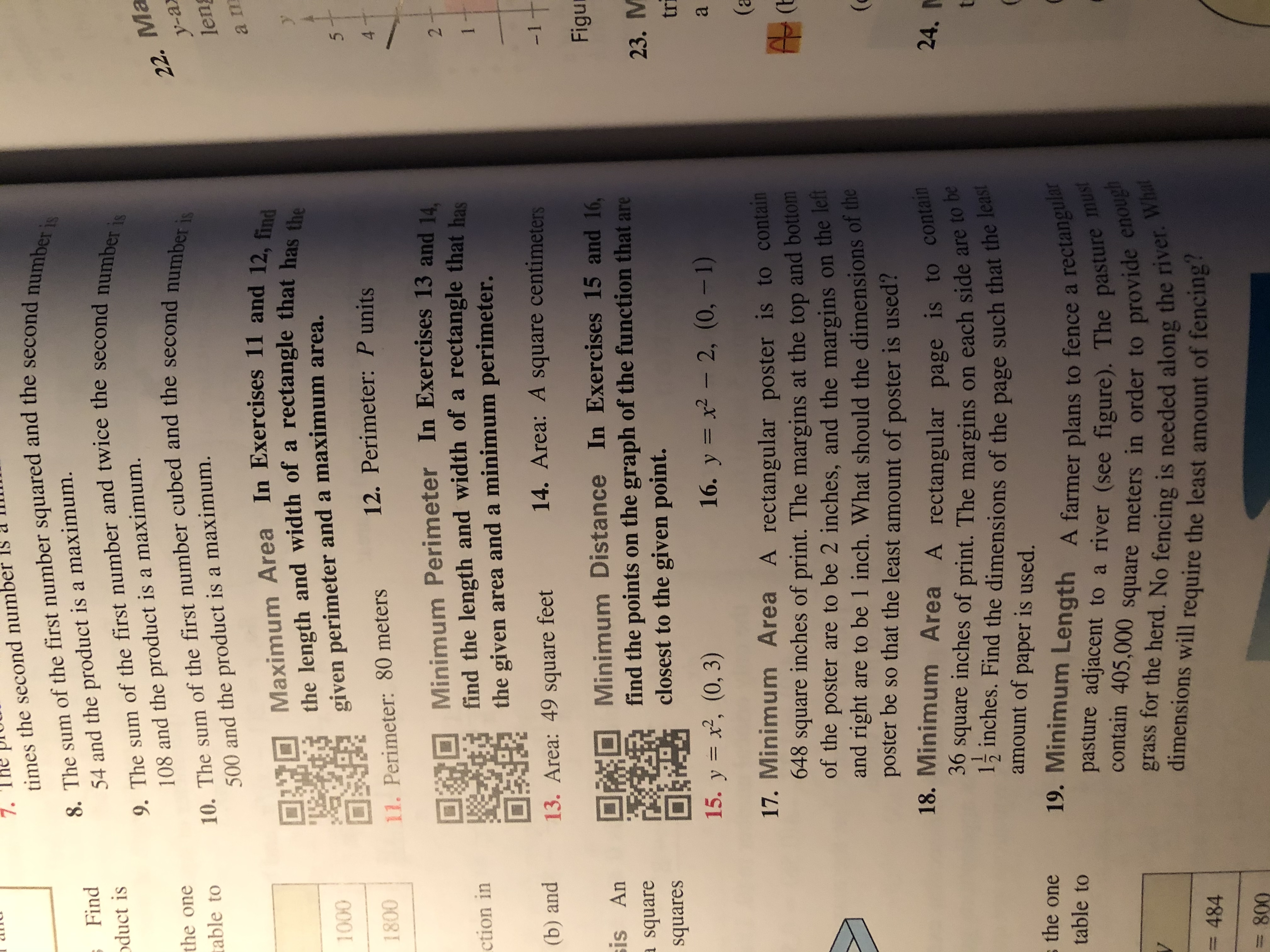# 7.times the second num8. The sum of the first number squared and the second number is9. The sum of the first number and twice the second number is10. The sum of the first number cubed and the second number issFindoduct is54 and the product is a maximum.22. Маy-a108 and the product is a maximum.the onetable to500 and the product is a maximum.lenMaximum Area In Exercises 11 and 12, findthe length and width of a rectangle that has thegiven perimeter and a maximum area.a m1000512. Perimeter: P units180011. Perimeter: 80 meters4Minimum Perimeter In Exercises 13 and 14find the length and width of a rectangle that hasthe given area and a minimum perimeter.2ction in(b) and14. Area: A square centimeters13. Area: 49 square feet-11FiguMinimum Distance In Exercises 15 and 16,find the points on the graph of the function that areclosest to the given point.is Ansquare23. Мquarestri15. y 2, (0, 3)16. y x2- 2, (0, -1)a17. Minimum Area A rectangular poster is to contain648 square inches of print. The margins at the top and bottomof the poster are to be 2 inches, and the margins on the letand right are to be 1 inch. What should the dimensions of theposter be so that the least amount of poster is used?18. Minimum Area A rectangular page is to conta36 square inches of print. The margins on each side are to be1inches. Find the dimensions of the page such that the least(a24.tamount of paper is used.s the one19. Minimum Length A farmer plans to fence a rectangularpasture adjacent to a river (see figure). The pasture mustcontain 405,000 square meters in order to provide enoughgrass for the herd. No fencing is needed along the river. Whattable toVdimensions will require the least amount of fencing?= 484800

Question
8 views

15help_outlineImage Transcriptionclose7. times the second num 8. The sum of the first number squared and the second number is 9. The sum of the first number and twice the second number is 10. The sum of the first number cubed and the second number is sFind oduct is 54 and the product is a maximum. 22. Ма y-a 108 and the product is a maximum. the one table to 500 and the product is a maximum. len Maximum Area In Exercises 11 and 12, find the length and width of a rectangle that has the given perimeter and a maximum area. a m 1000 5 12. Perimeter: P units 1800 11. Perimeter: 80 meters 4 Minimum Perimeter In Exercises 13 and 14 find the length and width of a rectangle that has the given area and a minimum perimeter. 2 ction in (b) and 14. Area: A square centimeters 13. Area: 49 square feet -11 Figu Minimum Distance In Exercises 15 and 16, find the points on the graph of the function that are closest to the given point. is An square 23. М quares tri 15. y 2, (0, 3) 16. y x2- 2, (0, -1) a 17. Minimum Area A rectangular poster is to contain 648 square inches of print. The margins at the top and bottom of the poster are to be 2 inches, and the margins on the let and right are to be 1 inch. What should the dimensions of the poster be so that the least amount of poster is used? 18. Minimum Area A rectangular page is to conta 36 square inches of print. The margins on each side are to be 1inches. Find the dimensions of the page such that the least (a 24. t amount of paper is used. s the one 19. Minimum Length A farmer plans to fence a rectangular pasture adjacent to a river (see figure). The pasture must contain 405,000 square meters in order to provide enough grass for the herd. No fencing is needed along the river. What table to V dimensions will require the least amount of fencing? = 484 800 fullscreen
check_circle

Step 1

The given function is y = x2 and the p...

### Want to see the full answer?

See Solution

#### Want to see this answer and more?

Solutions are written by subject experts who are available 24/7. Questions are typically answered within 1 hour.*

See Solution
*Response times may vary by subject and question.
Tagged in

### Calculus